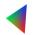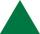# Area of RT 2

Calculate the area of right triangle whose legs have a length 5.8 cm and 5.8 cm.

Result

S =  16.82 cm2

#### Solution:Leave us a comment of example and its solution (i.e. if it is still somewhat unclear...):Be the first to comment!## Next similar examples:

1. Right triangleRight triangle legs has lengths 630 mm and 411 dm. Calculate the area of this triangle.
2. 22/7 circleCalculate approximately area of a circle with radius 20 cm. When calculating π use 22/7.
3. AnnulusThe radius of the larger circle is 8cm, the radius of smaller is 5cm. Calculate the contents of the annulus.
4. CableCable consists of 8 strands, each strand consists of 12 wires with diameter d = 0.5 mm. Calculate the cross-section of the cable.
5. 7 triangleThe triangle area is 26.7 cm2. Determine the side length l if appropriate height hl = 45.3 cm.
6. Area of a triangleWhat is the area of a triangle that has the base 4 1/4 and the height of 3 3/3?
7. Area of ditchHow great content area will have a section of trapezoidal ditch with a width of 1.6 meters above and below 0.57 meters? The depth of the ditch is 2.08 meters.
8. Triangle P2Can triangle have two right angles?
9. MidpointsTriangle whose sides are midpoints of sides of triangle ABC has a perimeter 45. How long is perimeter of triangle ABC?
10. QuizQAn isosceles triangle has two sides of length 7 km and 39 km. How long is a third side?
11. 3-bracket 3Two angles in a triangle are 90° and 60°. Has triangle at least two equal sides?
12. DisjointHow many elements have union and intersection of two disjoint sets when the first have 1 and secodn 8 elements.
13. AlleyAlley measured a meters. At the beginning and end are planted poplar. How many we must plant poplars to get the distance between the poplars 15 meters?
14. Find xSolve: if 2(x-1)=14, then x= (solve an equation with one unknown)
15. Dropped sheetsThree consecutive sheets dropped from the book. The sum of the numbers on the pages of the dropped sheets is 273. What number has the last page of the dropped sheets?
16. Simple equationSolve the following simple equation: 2. (4x + 3) = 2-5. (1-x)
17. RoundingThe following numbers round to the thousandth: